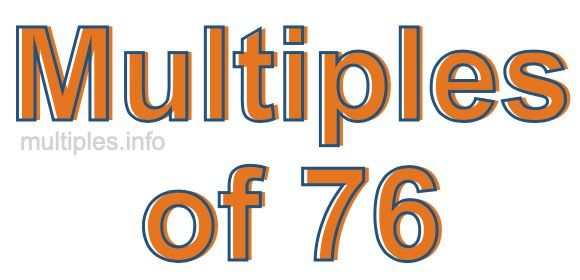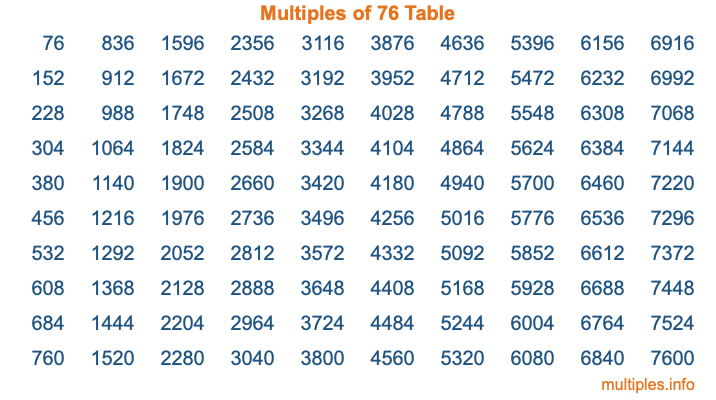Multiples of 76Welcome to the Multiples of 76 page. Here we will first teach you everything you will ever need to know about the multiples of 76, and then give you a study guide summary of everything we taught you to make sure you remember it all. Use this page to look up facts and learn information about the multiples of 76. This page will make you a multiples of seventy-six expert!

Definition of Multiples of 76
Multiples of 76 are all the numbers that when divided by 76 equal an integer. Each of the multiples of 76 are called a multiple. A multiple of 76 is created by multiplying 76 by an integer.

Therefore, to create a list of multiples of 76, you start with 1 multiplied by 76, then 2 multiplied by 76, then 3 multiplied by 76, and so on for as long as you want. Thus, the list of the first five multiples of 76 is 76, 152, 228, 304, and 380. To see a larger list of multiples of 76, see the printable image of Multiples of 76 further down on this page. We also have a category where you can choose any nth multiple of 76.

Multiples of 76 Checker
The Multiples of 76 Checker below checks to see if any number of your choice is a multiple of 76. In other words, it checks to see if there is any number (integer) that when multiplied by 76 will equal your number. To do that, we divide your number by 76. If the the quotient is an integer, then your number is a multiple of 76.

Is  a multiple of 76?

Least Common Multiple of 76 and ...
A Least Common Multiple (LCM) is the lowest multiple that two or more numbers have in common. This is also called the smallest common multiple or lowest common multiple and is useful to know when you are adding our subtracting fractions. Enter one or more numbers below (76 is already entered) to find the LCM.

Check out our LCM Calculator if you need more details about the Least Common Multiple or if you need the LCM for different numbers for adding and subtraction fractions.

nth Multiple of 76
As we stated above, 76 is the first multiple of 76, 152 is the second multiple of 76, 228 is the third multiple of 76, and so on. Enter a number below to find the nth multiple of 76.

th multiple of 76

Multiples of 76 vs Factors of 76
76 is a multiple of 76 and a factor of 76, but that is where the similarities end. All postive multiples of 76 are 76 or greater than 76. All positive factors of 76 are 76 or less than 76.

Below is the beginning list of multiples of 76 and the factors of 76 so you can compare:

Multiples of 76: 76, 152, 228, 304, 380, etc.

Factors of 76: 1, 2, 4, 19, 38, 76

As you can see, the multiples of 76 are all the numbers that you can divide by 76 to get a whole number. The factors of 76, on the other hand, are all the whole numbers that you can multiply by another whole number to get 76.

It's also interesting to note that if a number (x) is a factor of 76, then 76 will also be a multiple of that number (x).

Multiples of 76 vs Divisors of 76
The divisors of 76 are all the integers that 76 can be divided by evenly. Below is a list of the divisors of 76.

Divisors of 76: 1, 2, 4, 19, 38, 76

The interesting thing to note here is that if you take any multiple of 76 and divide it by a divisor of 76, you will see that the quotient is an integer.

Multiples of 76 Table
Below is an image of the first 100 multiples of 76 in a table. The table is in chronological order, column by column. The first column has the first ten multiples of 76, the second column has the next ten multiples of 76, and so on.The Multiples of 76 Table is also referred to as the 76 Times Table or Times Table of 76. You are welcome to print out our table for your studies.

Negative Multiples of 76
Although not often discussed or needed in math, it is worth mentioning that you can make a list of negative multiples of 76 by multiplying 76 by -1, then by -2, then by -3, and so on, to get the following list of negative multiples of 76:

-76, -152, -228, -304, -380, etc.

Multiples of 76 Summary
Below is a summary of important Multiples of 76 facts that we have discussed on this page. To retain the knowledge on this page, we recommend that you read through the summary and explain to yourself or a study partner why they hold true.

There are an infinite number of multiples of 76.

A multiple of 76 divided by 76 will equal a whole number.

76 divided by a factor of 76 equals a divisor of 76.

The nth multiple of 76 is n times 76.

The largest factor of 76 is equal to the first positive multiple of 76.

76 is a multiple of every factor of 76.

76 is a multiple of 76.

A multiple of 76 divided by a divisor of 76 equals an integer.

76 divided by a divisor of 76 equals a factor of 76.

Any integer times 76 will equal a multiple of 76.

Multiples of a Number
Here you can get the multiples of another number, all with the same attention to detail as we did for multiples of 76 on this page.

Multiples of
Multiples of 77
Did you find our page about multiples of seventy-six educational? Do you want more knowledge? Check out the multiples of the next number on our list!